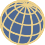## Computer Interfaces Transform Mathematical StudiesTypical geometric problems of interest to mathematical visualization applications involve both static structures, such as real or complex manifolds, and changing structures requiring interactions, such as the moves that transform one knot into an equivalent knot. Our goal is to show the nature of the interrelationship between mathematics and computer science, especially computer graphics, computer haptics, and multitouch technologies. We adopt for the most part a computer scientist’s perspective on the progress, techniques, and prospects of mathematical visualization, emphasizing those areas of 3D and 4D geometry where interactive paradigms are of growing importance. Here, we present a selection of three computer interfaces by which we may push our visual understanding of relatively simple geometric objects as far as our imagination can take us.